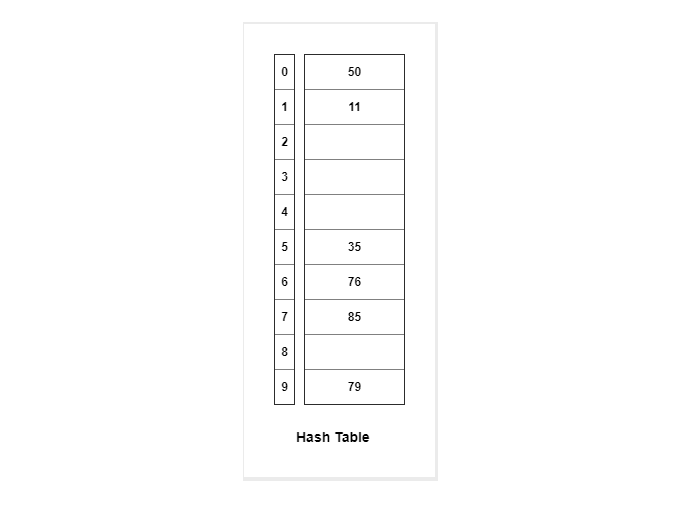# Hash Functions and Hash Tables

Hashing is the process of generating a value from a text or a list of numbers using a mathematical function known as a hash function.There are many hash functions that use numeric numeric or alphanumeric keys. Different hash functions are given below:

## Hash Functions

The following are some of the Hash Functions −

### Division Method

This is the easiest method to create a hash function. The hash function can be described as −

h(k) = k mod n

Here, h(k) is the hash value obtained by dividing the key value k by size of hash table n using the remainder. It is best that n is a prime number as that makes sure the keys are distributed with more uniformity.

An example of the Division Method is as follows −

k=1276
n=10
h(1276) = 1276 mod 10
= 6

The hash value obtained is 6

A disadvantage of the division method id that consecutive keys map to consecutive hash values in the hash table. This leads to a poor performance.

### Multiplication Method

The hash function used for the multiplication method is −

h(k) = floor( n( kA mod 1 ) )

Here, k is the key and A can be any constant value between 0 and 1. Both k and A are multiplied and their fractional part is separated. This is then multiplied with n to get the hash value.

An example of the Multiplication Method is as follows −

k=123
n=100
A=0.618033
h(123) = 100 (123 * 0.618033 mod 1)
= 100 (76.018059 mod 1)
= 100 (0.018059)
= 1

The hash value obtained is 1

An advantage of the multiplication method is that it can work with any value of A, although some values are believed to be better than others.

### Mid Square Method

The mid square method is a very good hash function. It involves squaring the value of the key and then extracting the middle r digits as the hash value. The value of r can be decided according to the size of the hash table.

An example of the Mid Square Method is as follows −

Suppose the hash table has 100 memory locations. So r=2 because two digits are required to map the key to memory location.

k = 50
k*k = 2500
h(50) = 50

The hash value obtained is 50

## Hash Tables

A hash table is a data structure that maps keys to values. It uses a hash function to calculate the index for the data key and the key is stored in the index.

An example of a hash table is as follows −

The key sequence that needs to be stored in the hash table is −

35 50 11 79 76 85

The hash function h(k) used is:

h(k) = k mod 10

Using linear probing, the values are stored in the hash table as −